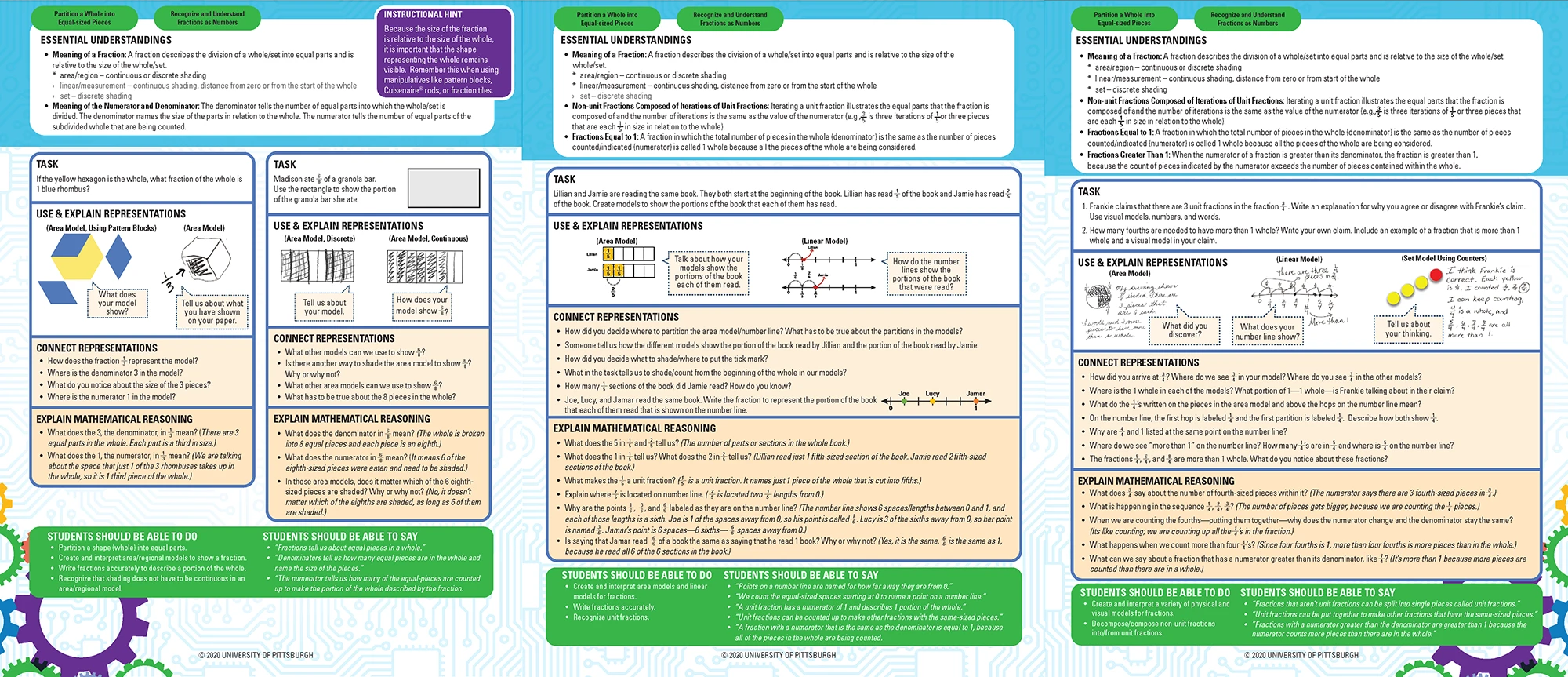# Math Planning Essentials

Each Math Planning Essentials guide (MPE) pulls together key elements of lesson planning that are organized by a set of essential understandings—the underlying mathematical truths worth exploring for a given concept of study.

MPEs can help make sense of these truths by providing information about how they might play out as students’ conceptual understanding develops. Each MPE provides:

• Essential Understandings that name what students need to know for a mathematical concept.
• Tasks to contextualize the mathematics.
• Ways students may Use & Explain Representations in response to the tasks, paired with suggestions to further elicit student thinking.
• Prompts and questions that
• ask students to Make Connections among different representations shown in and across student responses and
• require students to Explain Mathematical Reasoning about the ideas articulated in the essential understandings.
• A generalized list of what Students Should be Able to Do and Say, if they have made sense of the essential understandings.Sample of Understanding Fractions as Numbers.